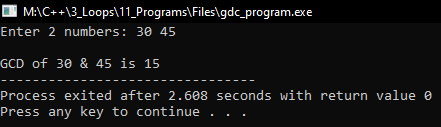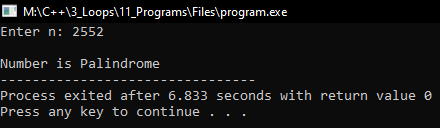# Programs using Loops in C++

## Programs using Loops in C++

In this article, I am going to discuss Programs using Loops in C++ with Examples. Please read our previous articles, where we discussed Armstrong Number using Loop in C++ with Examples.

##### Program to find GCD of two numbers using C++:

GCD means ‘Greatest Common Division’. It is the largest number that divides both of them. Let us look at the program.

```#include <iostream>
using namespace std;
int main()
{
int n, m;
cout << "Enter 2 numbers: ";
cin >> m >> n;
cout << endl;
cout << "GCD of " << m << " & " << n << " is ";
while (m != n)
{
if (m > n)
m = m - n;
else if (n > m)
n = n - m;
}
cout << m;
return 0;
}
```
###### Output:##### Program to check given number is palindrome or not using C++:

When the given number is equal to its reverse then it is called palindrome i.e. 151, 2552, etc. Let us look at the program.

```#include <iostream>
using namespace std;
int main ()
{
int n, r, rev = 0, m;
cout << "Enter n: ";
cin >> n;
cout << endl;
m = n;
while (n > 0)
{
r = n % 10;
n = n / 10;
rev = rev * 10 + r;
}
cout << "Number is ";
if (rev == m)
cout << "Palindrome";
else
cout << "not Palindrome";
return 0;
}
```
###### Output: